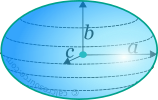## Properties of Ellipsoid

This tool calculates the basic geometric properties of an ellipsoid. Enter the shape dimensions a, b and c below. The calculated results will have the same units as your input. Please use consistent units for any input.

 a = b = c =
 Geometric properties: Volume = Surface area =## Definitions

### Geometry

The ellipsoid is a 3-dimensional surface, the points of which, in Cartesian coordinates, satisfy the following equation:

where a,b,c are the radii of the ellipsoid along its principal axes x, y and z respectively. It can be seen from the above formula, that ellipsoid is, in three dimensions, analogous to the ellipse in two dimensions.

The volume enclosed by an ellipsoid is given by the formula:

where a, b, c the three radii along the ellipsoid principal axes.